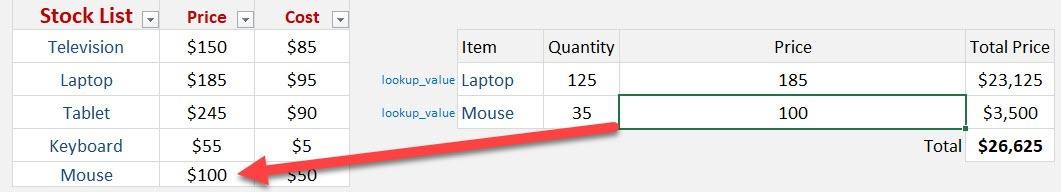What does it do?

Searches for a value in the first column of a table array and returns a value in the same row from another column (to the right) in the table array.

Formula breakdown:

=VLOOKUP(lookup_value, table_array, col_index_num, [range_lookup])

What it means:

=VLOOKUP(this value, TableName, and get me value in this column, Exact Match/FALSE/0])

Excel Tables are just amazing and should be used all the time, whether you have 2 rows or 200,000 rows of data!

You can read the benefits of using an Excel Table here:

Excel Tables

When you use a Vlookup formula to lookup in an Excel Table then your formula becomes dynamic due to its structured referencing.

What that means is that as the Excel Table expands with more data added to it, your Vlookup formula’s 2nd argument (table_array) does not need to be updated as it refers to the Excel Table as a whole by referring to its name eg Table1  or Table2  or Table3  etc

In the example below our Excel Table name is Table2 and as we add more rows of data to it, the Vlookup formula does not need to be adjusted.  How bloody cool is that?

STEP 1: We need to convert the data into an Excel Table. Press Ctrl + T then press OK.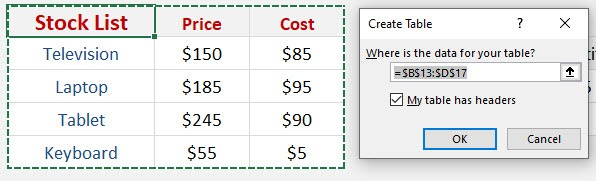STEP 2: Now let us create the formula to get the price of the Laptop. Let us use the VLOOKUP formula:

## =VLOOKUP(G15, Table1, 2, FALSE)

This will get the lookup value (Laptop in Cell G15), then search in the first column of Table1.

Afterwards it will get the value in Column #2 which is the price. The FALSE means is we want to get the exact match.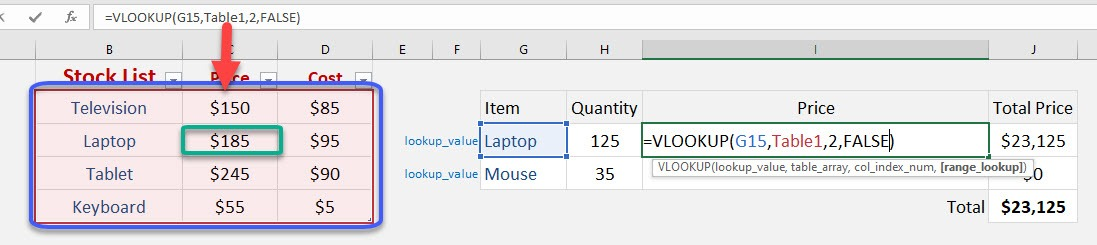STEP 3: Drag down the formula to copy it across the table. Notice that the second row is looking for the price of Mouse. This does not exist in our data table yet.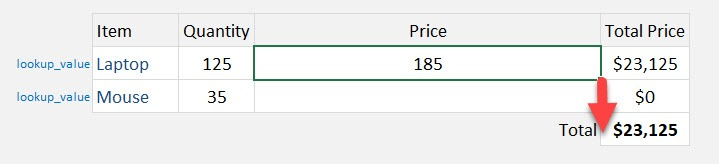STEP 4: Now add and type in a new row in our table for the price of the mouse.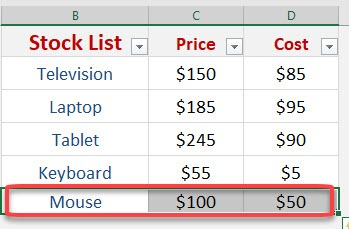The beauty with this is our VLOOKUP formula still works fine. Since we are using the Table1, there is no need to update the range of values that our VLOOKUP will use. It is now automatically included and the price of the mouse is retrieved right away.